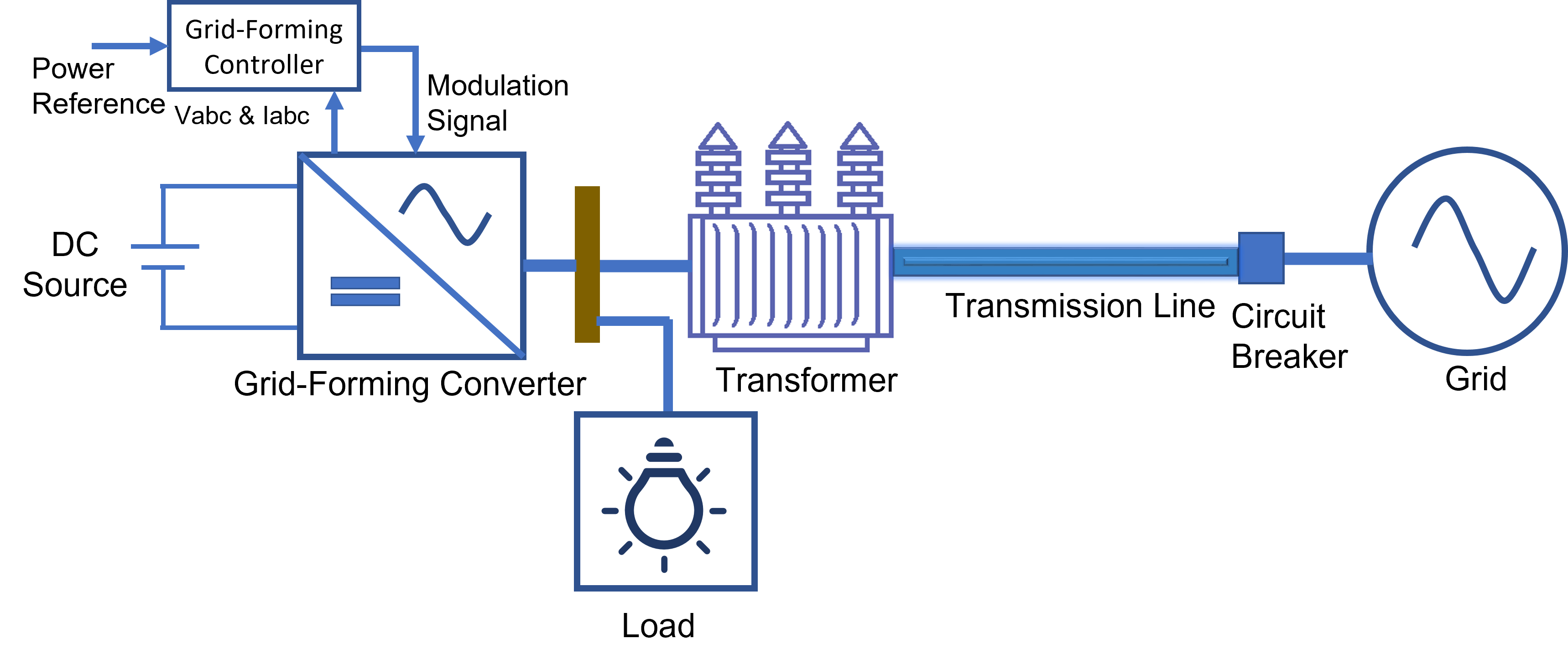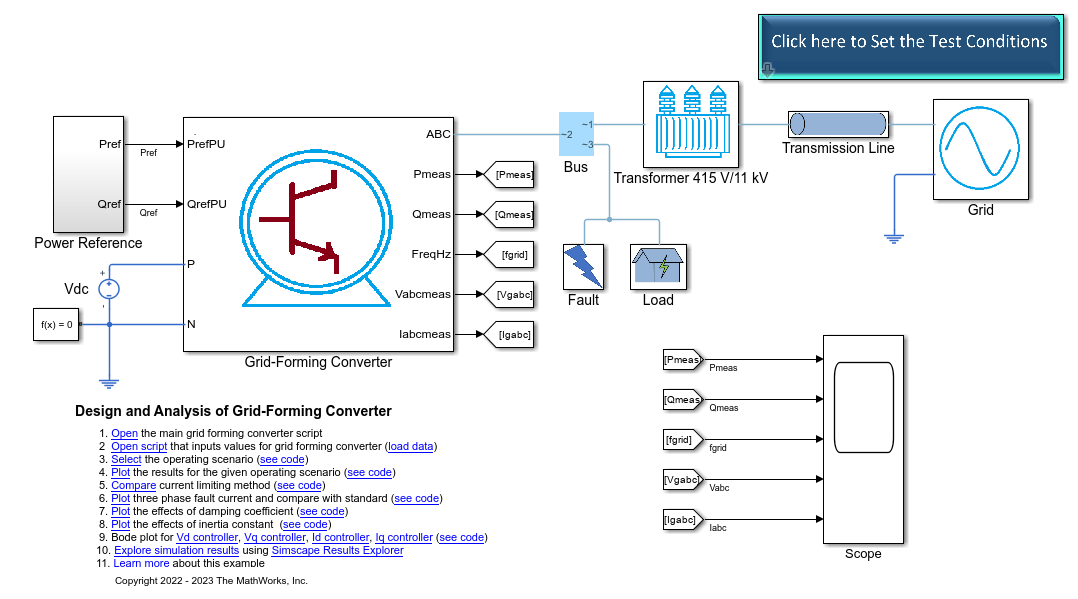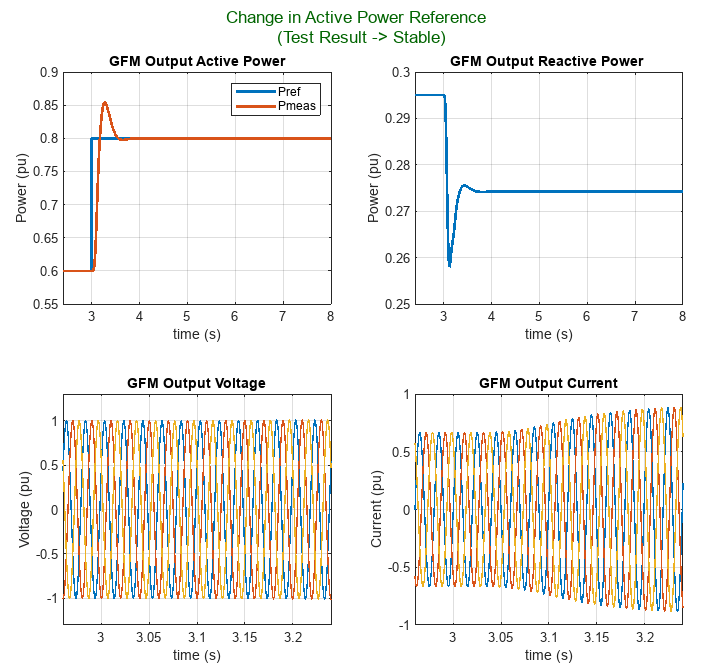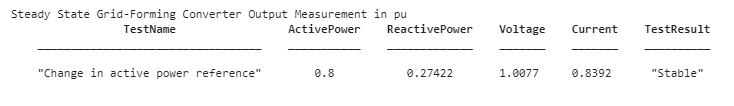# Design and Analyze Grid-Forming Converter

Since R2023b

This example shows how to design and analyze the performance of a grid-forming (GFM) converter under 13 predefined test scenarios. You can then compare the test results to the grid code standards to ensure desiderable operation and compliance. The GFM converter in this example provides an alternative inertia emulation technique, configurable control loops, different current limiting methods, and is suitable for a wide range of network strengths. You can download this model in MATLAB® or access it from MATLAB Central File Exchange and GitHub®.

### Example Overview

In this example, you learn how to:

• Identify the performance requirements from the grid code standard.

• Design a grid-forming controller using droop control and virtual synchronous machine control.

• Design different current limitation strategies using virtual impedance and current saturation methods.

• Verify the grid-forming technical specifications from grid operators for changes in voltage, frequency, and phase.

• Evaluate the system performance against changes in inertia, damping, and faults.Clone the up-to-date repository in the current folder using the `gitclone` function.

`gitclone("https://github.com/simscape/Power-Converter-Circuit-Control-Simscape");`

### Open Project

After you use the `gitclone` function, MATLAB creates a new folder in the current folder. This example uses projects to manage the supporting files. Open the `GridFormingConverterWithSimscape` project file. If you have any projects open, MATLAB closes them before loading this project. Configuring the project environment takes several minutes because the model has hundreds of supporting files. The project opens an overview in your web browser that helps you explore the model.

`openProject("Power-Converter-Circuit-Control-Simscape");`

### Explore Project

#### GFM Converter System

The top-level model shows the design of the GFM converter system in this example. The model comprises a 500 kVA, 415 V, 50 Hz, three-phase GFM converter that connects to a 415 V/11 kV step-up transformer. A transmission line connects the transformer to an 11 kV grid. A local load connects to the GFM converter output at the low-voltage level. The grid-forming converter model provides the test harness that allows you to change the grid voltage, frequency, phase, local load, short-circuit ratio (SCR), X/R ratio, power reference, islanding mode, and apply faults.#### Controller Strategy

To specify the control strategy, set the value of the `testCondition.activePowerMethod` variable to `Virtual Synchronous Machine` or `Droop Control`. To select the virtual synchronous machine (VSM) strategy, at the MATLAB® Command Window, run:

```testCondition.activePowerMethod = 'Virtual Synchronous Machine'; ```

The VSM control method mimics the synchronous machine inertia and damping. The software computes the controller damping power by using either a constant frequency reference or a measured grid system frequency. The damping power based on the grid frequency measurement offers better performance during the grid frequency disturbances.

#### Fault Ride-Through Method

For grid code standards, all inverter-based resources must have a ride-through capability for voltage and frequency disturbances. To specify the fault ride-through option, set the value of the `testCondition.currentLimitMethod` variable to either:

• `Virtual Impedance`

• `Current Limiting`

• `Virtual Impedance and Current Limiting`

To set the fault ride-through option to `Virtual Impedance`, at the MATLAB® Command Window, run:

```testCondition.currentLimitMethod = 'Virtual Impedance'; ```

#### Test Scenarios

This example provides 13 test scenarios under which you can evaluate the performance of the GFM converter and controllers:

• Normal operation — Simulate the model without any disturbance.

• Change in active power reference — Instantaneous change in the active power reference.

• Change in reactive power reference — Instantaneous change in the reactive power reference.

• Change in grid voltage — Instantaneous change in the grid voltage.

• Change in local load — Instantaneous change in the local load.

• Small change in grid frequency (0.5 Hz) — Increase the system frequency by 0.5 Hz at the rate of 1 Hz/s.

• Large change in grid frequency (2 Hz) — Increase the system frequency by 2 Hz at the rate of 2 Hz/s.

• Full range change in grid frequency — Increase the system frequency by 2 Hz at the rate of 2 Hz/s, wait for 5 seconds, and then decrease the frequency by 5 Hz at the rate of 1 Hz/s.

• Change in grid phase by 10 degrees — Apply an instantaneous change in the phase by 10 degrees.

• Change in grid phase by 60 degrees — Apply an instantaneous change in the phase by 60 degrees.

• Permanent three-phase fault — Generate a three-phase-to-ground fault and measure the reactive power.

• Temporary three-phase fault — Generate a three-phase-to-ground fault and clear it after a specific amount of time.

• Islanding operation — During steady-state operation, the grid circuit breaker trips to simulate the GFM converter at the islanded condition.

Use the `PlotGridFormingConverter` function to plot the simulation results for the 13 test scenarios. This function also analyzes the measured active power and frequency to check if the system reaches a stable state at the end of the simulation time. The `PlotGridFormingConverter` function takes these inputs:

• `testCondition` — Simulation settings, specified as a `struct` of five variables.

• `plotFlag` — Option to plot the results and a summary MATLAB table. To plot the results, set this input to 1.

• Optional third variable — Set this to `"All"` to simulate all the test scenarios at once.

You can define the test scenario and the test variable profile with time in the `GridFormingConverterTestCondition.mlx` file.

#### Simulate Test Scenario

To setup and simulate a test scenario, first set the values for the `testCondition` variable.

The `testCondition` variable defines:

• Active power method

• Current limiting method

• Grid short-circuit ratio

• Grid X/R ratio

• Test name

This code sets the active power control method of the GFM converter to `"Virtual Synchronous Machine"`, the fault ride-through method to `"Virtual Impedance"` with a grid short-circuit ratio of 2.5 and a X/R ratio of 5.

In this test scenario, the system experiences an instantaneous change in the active power reference. This test scenario allows you to study the GFM converter tracking ability, response time, and output voltage fluctuations. To set and simulate this test scenario, at the MATLAB Command Window, run:

```testCondition.activePowerMethod = 'Virtual Synchronous Machine'; testCondition.currentLimitMethod = 'Virtual Impedance'; testCondition.SCR = 2.5; testCondition.XbyR = 5; testCondition.testCondition = 'Change in active power reference'; outputTable = PlotGridFormingConverter(testCondition,1); ```To find the latest examples from the MathWorks Simscape Team, see MathWorks Simscape Team on MATLAB Central.